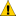The Select ParserSelect parsers may be used to identify a single parser from a given list of parsers, which successfully recognizes the current input sequence. Example:

```    rule<> rule_select =
select_p
(
parser_a
, parser_b
/* ... */
, parser_n
);```

The parsers (parser_a, parser_b etc.) are tried sequentially from left to right until a parser matches the current input sequence. If there is a matching parser found, the select_p parser returns the parser's position (zero based index). For instance, in the example above, 1 is returned if parser_b matches.

There are two predefined parsers of the select parser family: select_p and select_fail_p. These parsers differ in the way the no match case is handled (when none of the parsers match the current input sequence). While the select_p parser will return -1 if no matching parser is found, the select_fail_p parser will not match at all.

The following sample shows how the select parser may be used very conveniently in conjunction with a switch parser:

```    int choice = -1;
rule<> rule_select =
select_fail_p('a', 'b', 'c', 'd')[assign_a(choice)]
>>  switch_p(var(choice))
[                case_p<0>(int_p),                case_p<1>(ch_p(',')),                case_p<2>(str_p("bcd")),                default_p            ]        ;```

This example shows a rule, which matches:

• 'a' followed by an integer
• 'b' followed by a ','
• 'c' followed by "bcd"
• a single 'd'.

For other input sequences the give rule does not match at all.BOOST_SPIRIT_SELECT_LIMIT The number of possible entries inside the select_p parser is limited by the Spirit compile time constant BOOST_SPIRIT_SELECT_LIMIT, which defaults to 3. This value should not be greater than the compile time constant given by PHOENIX_LIMIT (see phoenix). Example: // Define these before including anything else #define PHOENIX_LIMIT 10 #define BOOST_SPIRIT_SELECT_LIMIT 10